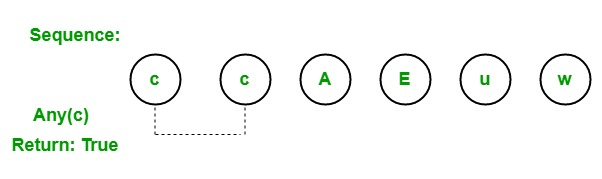Related Articles

# LINQ | Quantifier Operator | Any

• Last Updated : 26 May, 2019

In LINQ, quantifier operators are used to returning a boolean value which shows that whether some or all elements satisfies the given condition. The Standard Query Operator supports 3 different types of quantifier operators:

1. All
2. Any
3. Contains

#### Any Operator

The Any operator is used to check whether any element in the sequence or collection satisfy the given condition. If one or more element satisfies the given condition, then it will return true. If any element does not satisfy the given condition, then it will return false.• It is overloaded in two different types:
• Any<TSource>(IEnumerable<TSource>): This method is used to check whether a sequence contains any elements.
• Any<TSource>(IEnumerable<TSource>, Func<TSource, Boolean>): This method is used to check whether any element of a sequence satisfies a condition.
• It is generally used with where clause.
• It does not support query syntax in C# and VB.Net languages.
• It support method syntax in both C# and VB.Net languages.
• It present in both the Queryable and Enumerable class.
• It will throw ArgumentNullException if the given source is null.
• It does not return value instead of this it return true or false.
• The return type of this operator is System.Boolean.

Example 1:

 `// C# program to illustrate the``// use of Any operator``using` `System;``using` `System.Linq;``using` `System.Collections.Generic;`` ` `class` `GFG {`` ` `    ``// Main Method``    ``static` `public` `void` `Main()``    ``{`` ` `        ``// Data source``        ``int``[] sequence1 = {34, 56, 77, 88,``                          ``99, 10, 23, 46};``                           ` `        ``string``[] sequence2 = {``"aa"``, ``"oo"``, ``"gg"``,``                             ``"ww"``, ``"jj"``, ``"uu"``};``                              ` `        ``char``[] sequence3 = {``'c'``, ``'c'``, ``'A'``,``                                ``'E'``, ``'u'``};`` ` `        ``// Check the sequence1 if it ``        ``// contain any element as 10``        ``// Using Any operator``        ``var` `result1 = sequence1.Any(seq => seq == 10);`` ` `        ``Console.WriteLine(``"Is the given sequence "``+``           ``"contain element as 10 : {0}"``, result1);`` ` `        ``// Check the sequence2 if it ``        ``// contain any element as "oo"``        ``// Using Any operator``        ``var` `result2 = sequence2.Any(seq => seq == ``"oo"``);`` ` `        ``Console.WriteLine(``"Is the given sequence "``+``          ``"contain element as 'oo' : {0}"``, result2);`` ` `        ``// Check the sequence3 if it ``        ``// contain any element as 'c'``        ``// Using Any operator``        ``var` `result3 = sequence3.Any(seq => seq == ``'c'``);`` ` `        ``Console.WriteLine(``"Is the given sequence "``+``          ``"contain element as 'c' : {0}"``, result3);``    ``}``}`
Output:
```Is the given sequence contain element as 10 : True
Is the given sequence contain element as 'oo' : True
Is the given sequence contain element as 'c' : True
```

Example 2:

 `// C# program to check the list ``// contain employee data or not``using` `System;``using` `System.Linq;``using` `System.Collections.Generic;`` ` `// Employee details``public` `class` `Employee { }`` ` `class` `GFG {`` ` `    ``// Main method``    ``static` `public` `void` `Main()``    ``{``        ``List emp = ``new` `List() { };`` ` `        ``// Query to check this list ``        ``// contain employee data or not``        ``// Using Any operator``        ``var` `res = emp.Any();``         ` `        ``Console.WriteLine(``"Is the list contain "``+``                    ``"employees data?: {0}"``, res);``    ``}``}`
Output:
```Is the list contain employees data?: False
```

My Personal Notes arrow_drop_up